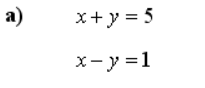# System of linear equations, find x,y

Hi my question is pure mathematical problem, this is my code that finds x and y of System of linear equations. In this case, for this easy example equation:My question is- How can I transform equations like these to from Ax - b = 0 and then find x,y with Pytorch

Equations like these to from Ax - b = 0

``````x = torch.nn.Parameter (torch.zeros (2))
print(x.shape)
print(x)

def model(x):
k = x + x -5
z = x - x -1

p =  torch.tensor([k, z])
return k,z

for i in range(500):
target = torch.nn.Parameter (torch.zeros (2))

k,z = model(x)
loss = (0 - k)**2 + (0 - z)**2
print(loss,loss)
loss.backward()
optimizer.step()

print(x,x, "X Y ")

``````

I think if you understand the meaning of Ax=b it’s straightforward
Basically you have to figure out A and b
for a)
A=(1,1)
(1,-1)
and b = (5,1)
in the example
b) 2x+2=x-y → x+y=-2
then
A = (1,1)
(3,2)
b=(-2,0)

Obtaining A and b allow you to rewrite the problem in a matricial way reusing the code.

1 Like

Thank you I understand now